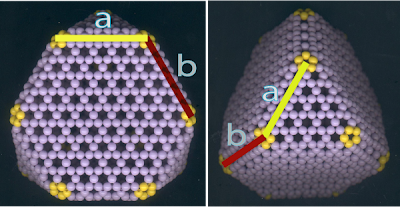## Friday, April 20, 2007

### Large Truncated Tetrahedral and Octahedral Fullerenes

Chuang Chern made this amazing truncated tetrahedron which consists of more than a thousand beads. I guess he probably spent more than 10 hours on this gigantic fullerene. This structure is essentially a natural extension of the system with T or Td symmetry as I shown before. All fullerenes belonging this catagory can be completely specified by two vectors, a and b. The first vector, a, denotes the relative arrangement among the three pentagons at each vertex as shown in the right figure below. When these three pentagons are not nearest neighbours with each other, the resulting tetrahedron becomes a truncated one, just like the one I have posted below.

The second vector, b, specifies the separation between two pentagons, belonging to two neighbouring vertices separately.## Approaching the Octahedral Limit

Now let's imagine we have a continuous Achimedean solid just like our truncated beaded tetrahedron which can be described by the same two vectors, a and b. Now let the vector b gradually changes to zero, then this tetrahedron will be continuously transformed into an octahedron. The following figure taken from Wikipedia demonstrates this can be done graphically.However, this is not possible in fullerene cages due to the intrinsic physical properties of the graphitic sheet. The smallest possible value of b is when these two pentagons are separated by one hexagon. We can't fuse two pentagons into one, the Euler theorem will be violated then. Additionally, since there are six vertices in an octahedron, every vertex of an octahedron must contain two pentagons. So the four faces around any one of the six vertices cannot be all the same. The two triangular faces lies along the direction defined by these two pentagons, the direction of vector b, will be different from the other two faces lying perpendicular to this direction. But when another vector, a, is very large, the truncated tetrahedron will approach the octahedron if the detailed structure around the vertices is ignored!

In general, it is not possible to have a fullerene with the octahedron symmetry, if only 5- and 6-member rings are allowed.